## Elements of Transfer Function Synthesis:

A transfer function is generally defined to specify the properties of a two port network. Hence Elements of Transfer Function Synthesis is also known as two port synthesis.

Consider a two port network as shown in the Fig. 7.26.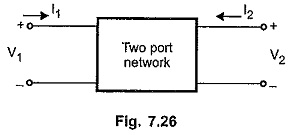V1 and I1 are voltage and current at port 1 while V2 and I2 are voltage and current at port 2.

Thus there are four variables associated with the network, the two are voltage variables V1, V2 and other two are current variables I1 and I2.

A ratio of voltage or current at one port to the voltage or current at the other port is called a transfer function of the network.

The following transfer functions can be defined for the two port network,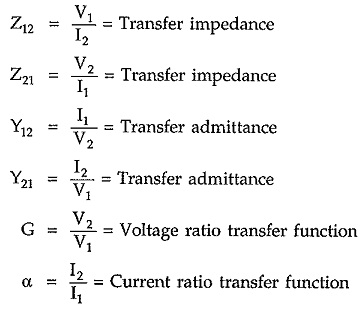A two port network is generally defined by its parameters such as z parameters and y parameters.

In the transfer function synthesis, the two port network is terminated at port 2 in resistance R. Let us express the transfer impedance and admittance functions of a two port terminated network, in terms of z and y parameters.

Note that the transfer functions are denoted by capital letters while the network parameters are denoted by small letters.

### Transfer Functions of Two Port Terminated Network:

Consider a two port network, terminated in resistance R as shown in the Fig. 7.27.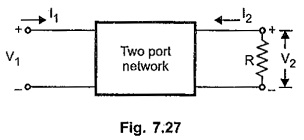Z21 = V2/V1 = Transfer impedance function

From the z parameters we can write,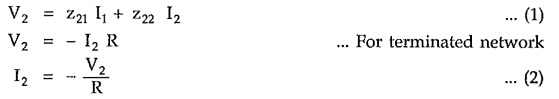Substituting in (1),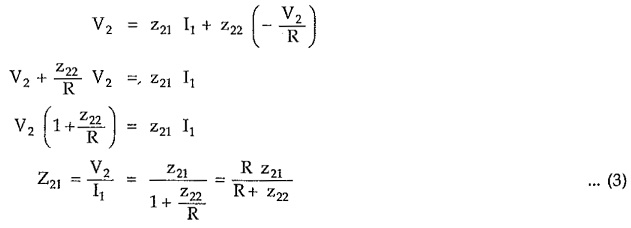The transfer impedance function Z21 is denoted as capital letter while z21, z22 are the z parameters, denoted by small letters.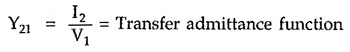Now

Y21 = I2/V1 = Transfer admittance function

From the y parameters we can write,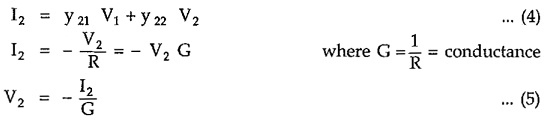Substituting in (4),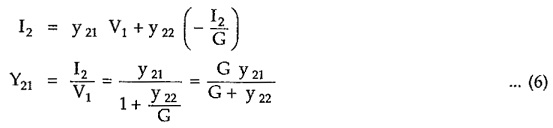Similar to impedance and admittance functions, the other transfer functions can also be expressed in z and y parameters.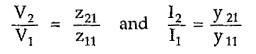The results are helpful in the synthesis of two port networks

### Properties of Transfer Functions:

The transfer function is the ratio of two polynomials in s.

It is denoted as T(s).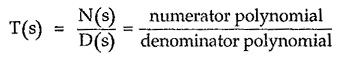The T(s) is the transfer function of linear network consisting of lumped bilateral passive elements. The properties of such T(s) are,

1. The T(s) is real when s is real.

When T(s) is rational with real coefficients, then this property gets satisfied.

1. The poles of T(s) are simple having zero or negative real parts. T(s) has no poles in right half of s plane.
2. The T(s) has no multiple poles on the jω axis.
3. The degree of polynomial N(s) can not exceed the degree of the polynomial D(s) by more than one.
4. The polynomial D(s) must be Hurwitz polynomial.
5. The T(s) can be split into odd and even parts as,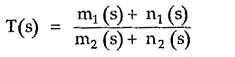where m1, m2 are even parts and n1, n2 are odd parts of N(s) and D(s) respectively.

Replacing s by jω we get,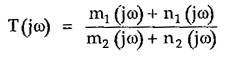From T(jω) the amplitude and phase response of T(s) can be obtained. The amplitude of T(jω) i.e. |T(jω)| is always even function of ω and amplitude response is symmetrical about y-axis.

If ∠ T(jω) = 0 for ω = 0 then the phase response is always an odd function of ω and the phase response is symmetrical about x-axis.

### Properties of Open Circuit and Short Circuit Parameters:

The specific properties of z and y parameters can be stated as,

1. The poles of the parameter z21 are also the poles of the parameters z11 and z22. But not all the poles of z11 and z22 are the poles of z21.
2. The poles of the parameter y21 are also the poles of the parameters y11 and y22 , But not all the poles of y11 and y22 are the poles of y21.
3. Let y11, y22 and y12 all have a pole at s = s1. Let k11 is the residue of y11, k22 is the residue of y22 and k12 is the residue of y11 at the pole s = s1.

Then for all the two port networks,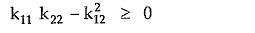This is called a residue condition.

Scroll to Top